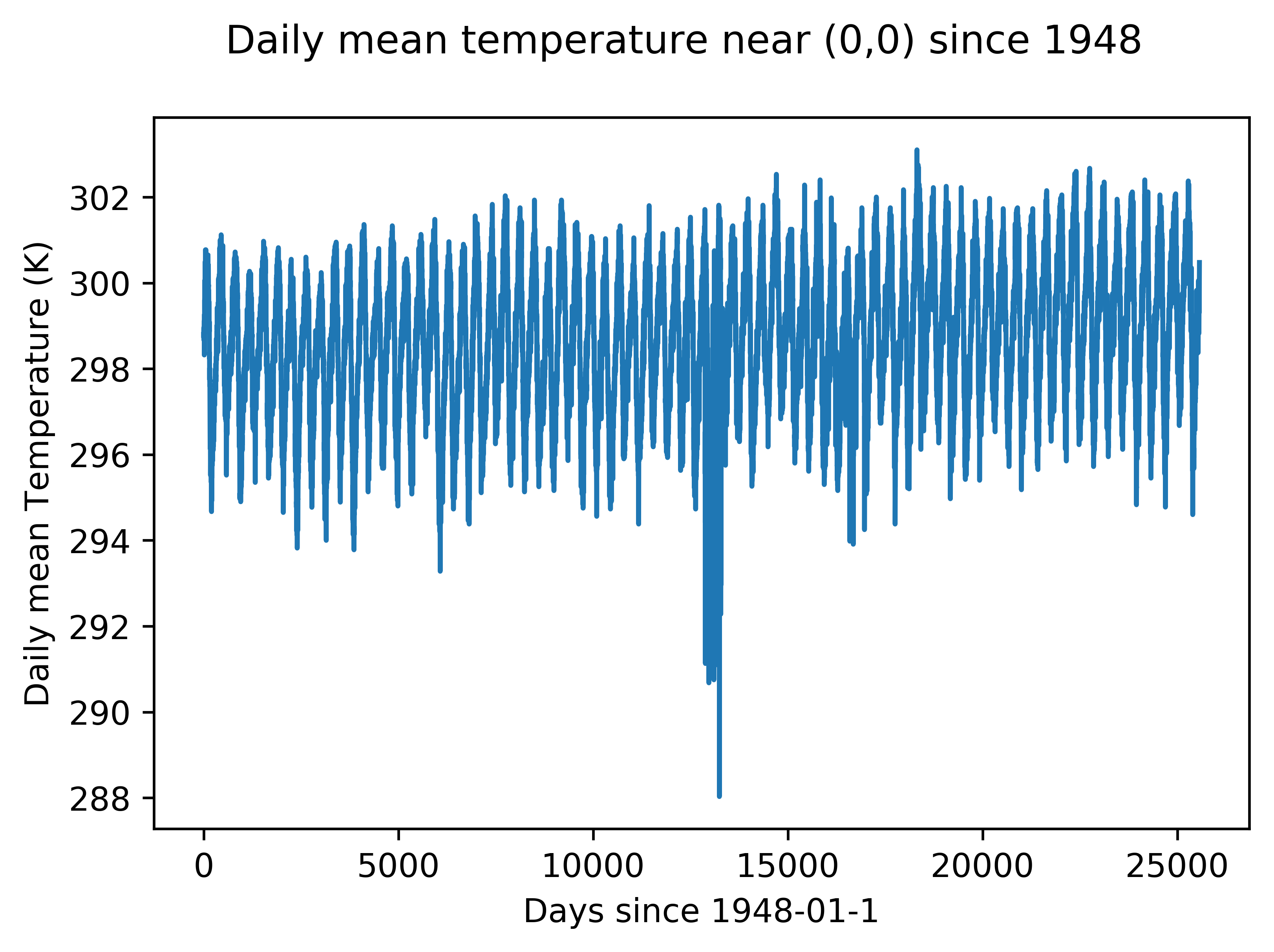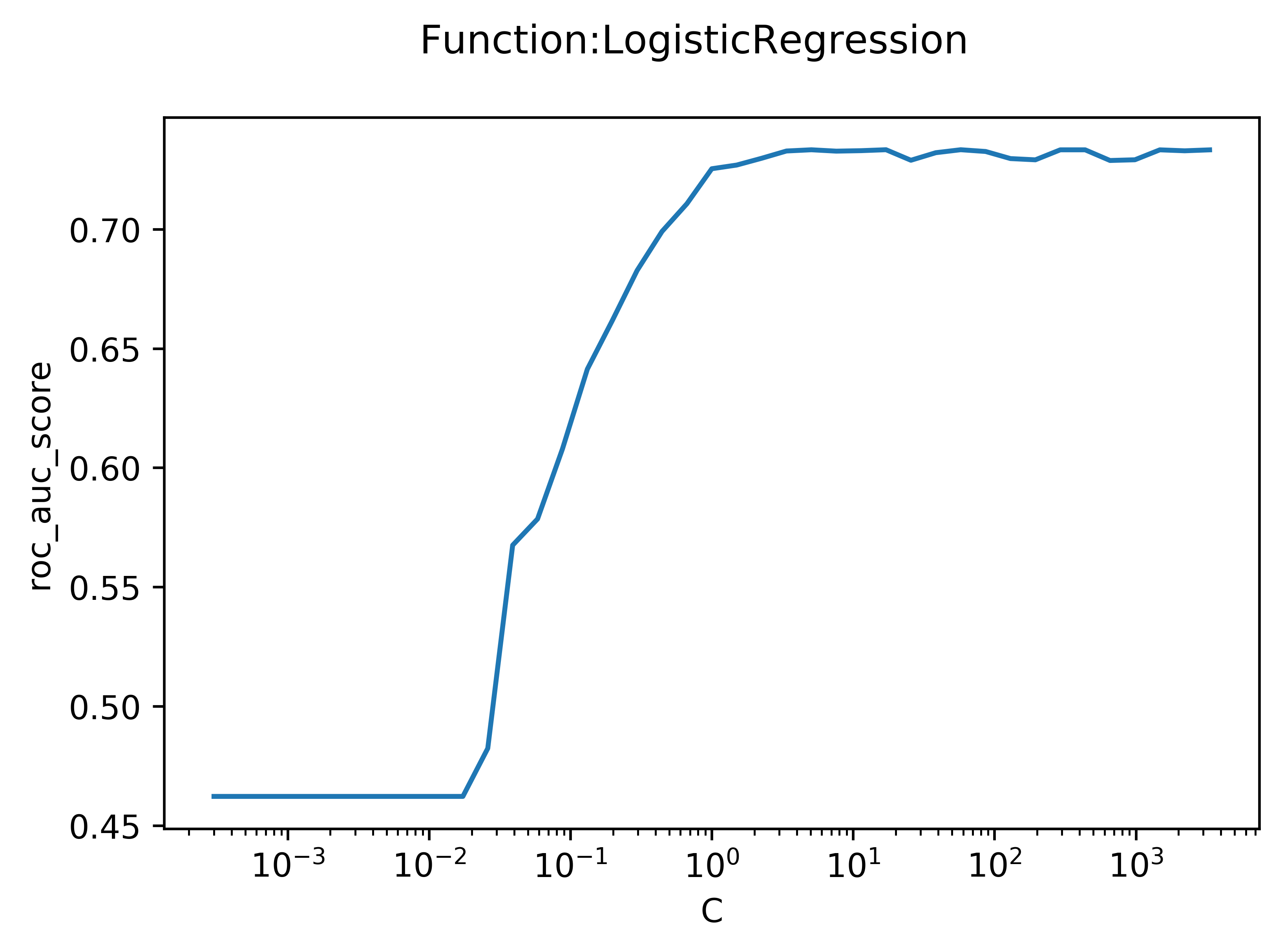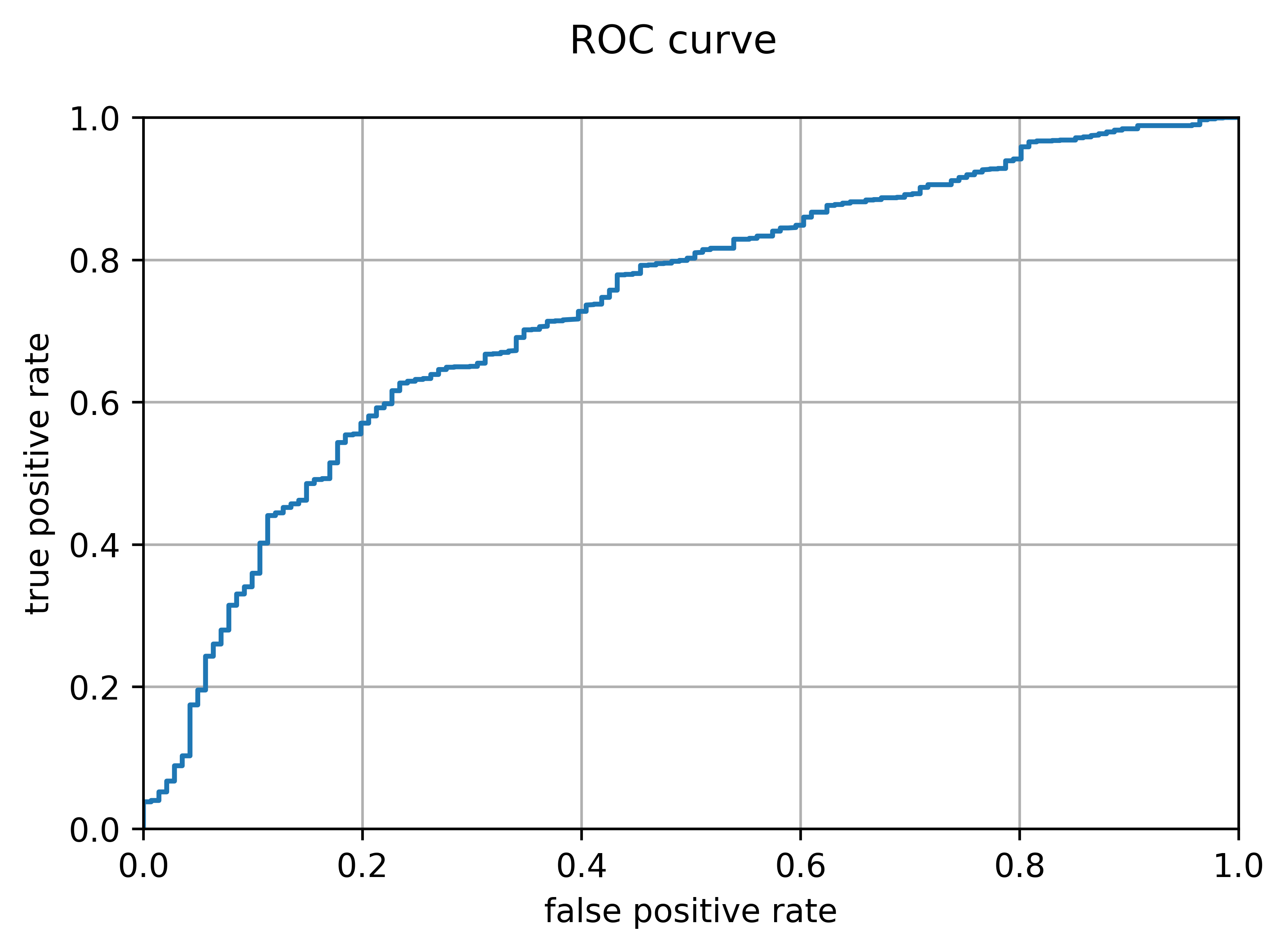# Research

## High school

I'm only getting into University-level research now, but here's some research I did in high school

### Can rocket failure be predicted with a statistical model?

Answer: Yes, but only somewhat well.

#### Demonstration of the data used

I used decades of data from NOAA to add the temperature variable to my model. Here is a demonstration of that data at an arbitrary point. An upwards trend in temperature is apparent.#### Hyperparameter tuning

I used a logistic regression model to predict whether a rocket launch would succeed ("1") or fail ("0"). To decrease the coefficiencts on noisy variables, a cost function was added. The plot seen below is the output of a function I wrote that fits an arbitrary model (in this case, Logistic Regression) repeatedly while varying an arbitrary hyperparameter (in this case, C, the weight of the cost function). A low C means that the cost function was weighted very heavily, and a high C represents a very low weight.#### ROC curve

Because different use-cases may necessitate different thresholds for deciding whether a rocket will fail or not, the model must be evaluated for every possible threshold. One method of doing this for a binary classifier is a receiver operating characteristic curve, which plots the true positive rate against the false positive rate as the threshold varies. Ideally, the area under the curve should be maximized (this area was the metric I used to choose a C value in the plot above). The plot below is the output of a function I wrote which generates and plots an ROC curve. It is evident that the model performs better than a coin toss (whose ROC curve would simply be a line x=y), but the area under the curve is not very large, at just 0.733.Here is the code I used for this research. It's not particularly high-quality or well-documented. It is intended to be run block-by-block in an IPython notebook.

`from datetime import date, timedeltaimport syssys.path.insert(0, '/home/aozerov/Dropbox/programming/jupyter')from netCDF4 import Datasetfrom ftplib import FTPfrom sklearn.linear_model import LogisticRegressionfrom sklearn.ensemble import RandomForestClassifierfrom sklearn.neighbors import KNeighborsClassifierfrom sklearn.tree import DecisionTreeClassifierfrom sklearn.ensemble import GradientBoostingClassifierfrom sklearn.svm import SVCfrom sklearn.neural_network import MLPClassifierfrom sklearn import treefrom sklearn.feature_selection import SelectKBestfrom sklearn.feature_selection import SelectPercentilefrom sklearn.feature_selection import f_classiffrom sklearn.feature_selection import chi2from sklearn.model_selection import train_test_splitfrom sklearn.preprocessing import Imputerfrom sklearn.metrics import f1_scorefrom sklearn.metrics import roc_auc_scorefrom sklearn.metrics import mean_squared_errorfrom sklearn.metrics import roc_curvefrom sklearn.metrics import confusion_matrixfrom IPython.display import clear_outputfrom IPython.display import SVG#NEED TO FIGURE OUT HOW TO MAKE THIS WORK:#from graphviz import Sourceimport pandas as pdimport numpy as npimport matplotlib.pyplot as pltimport osimport picklefrom sqlalchemy import create_engineimport funcsimport sql#auto import the funcs file%load_ext autoreload%autoreload 1%aimport funcs%aimport sqlfor i in range (1950, 2019):    ftp = FTP('ftp.cdc.noaa.gov')    ftp.login()    ftp.cwd('/Datasets/ncep.reanalysis.dailyavgs/surface')    file = open("[name-of-directory]/y%s.nc" %i, 'wb')    ftp.retrbinary('RETR air.sig995.%s.nc' %i, file.write)    ftp.quit()#run the resultant code printed by this in unix to concatenate all the new ncr filescommand = "ncrcat"for i in range(1948,2018):    command += " y"    command += str(i)    command += ".nc"command += " surface_temp.nc"print(command)os.environ["HDF5_USE_FILE_LOCKING"] = "FALSE"surface_temp = Dataset("/home/aozerov/Dropbox/programming/jupyter/mathia/surface_temp.nc", 'r', format='NETCDF4')lats = surface_temp.variables['lat'][:]lons = surface_temp.variables['lon'][:]print(lats)time = surface_temp.variables['time'][:]print(time)print(surface_temp.variables['time'][:])air = surface_temp.variables['air'][:]# Find the nearest latitude and longitude for 0,0location = {'name': '0,0', 'lat': 0, 'lon': 0}lat_idx = np.abs(lats - location['lat']).argmin()lon_idx = np.abs(lons - location['lon']).argmin()#CREDIT TO JONATHAN MCDOWELL FOR HIS LAUNCHLOG.#launchlog.csv is merely a slightly processed version of his log at:#https://planet4589.org/space/log/launchlog.txtlaunches = pd.read_csv("[path-to]/launchlog.csv")print(launches.head())print(launches.shape)print(launches.columns.values)launches = launches.rename(columns={'# Launch': 'launchnum'})launchesp = launches[launches.launchnum.notnull()]print(launchesp.shape)coldel = ['launchnum', 'Ref','Launch Date (UTC)', 'COSPAR', 'PL Name', 'Orig PL Name', 'SATCAT', 'LV S/N', 'Unnamed: 9', 'Unnamed: 12', 'Unnamed: 13', 'Unnamed: 14', 'Unnamed: 15', 'Unnamed: 17', 'Unnamed: 18', 'Unnamed: 19', 'Unnamed: 20', 'Unnamed: 21', 'Unnamed: 22']launchesp = launchesp.drop(columns = coldel)print(launchesp.columns.values)launchesp["surfacetemp"] = np.nanprint(time)timeadjust = []start = date(1800,1,1)for i in range (0, len(time)):    hours = time[i]    days = hours/24    delta = timedelta(days)     # Create a time delta object from the number of days    offset = start + delta    print(offset)    timeadjust.append(offset)    print(delta)#print temperature graph at 0,0print(np.arange(731))print(air[:, lat_idx, lon_idx].size)#np.column_stack((predictors_intercept, coefs_intercept))plt.figure(dpi=600)plt.plot(np.arange(air[:, lat_idx, lon_idx].size), air[:,lat_idx,lon_idx],'-')plt.suptitle('Daily mean temperature near (0,0) since 1948')plt.xlabel('Days since 1948-01-1')plt.ylabel('Daily mean Temperature (K)')plt.show()#CREDIT TO JONATHAN MCDOWELL FOR HIS LIST OF SITES.#sites.csv is merely a slightly processed version of his list at:#https://planet4589.org/space/lvdb/sdb/Sitessites = pd.read_csv("[path-to],sites2.csv")sites.head()# need to convert dates in launchesp to days*24 since 1948-01-01launch_surface_temps = []for i in range (0, launchesp.shape):    #conver 'LAUNCH DATE' column to date objects    ldatestr = launchesp.iloc[i]['LAUNCH DATE']    #ldateobj = datetime.strptime(ldatestr, '%Y-%m-%d').date()    ldateobj = date(*map(int, ldatestr.split('-')))    print(ldateobj)    sourcedate = date(1948,1,1)    tindex = (ldateobj-sourcedate).days    print(tindex)        sitestr = launchesp.iloc[i]['Site']    print(sitestr)    for j in range (0, sites.shape):        if sites.iloc[j]['code'] == sitestr:            lat = sites.iloc[j]['lat']            long = sites.iloc[j]['long']            break    lat_idx = np.abs(lats - lat).argmin()    lon_idx = np.abs(lons - long).argmin()    launch_surface_temp = air[tindex,lat_idx,lon_idx]    print(launch_surface_temp)    launch_surface_temps.append(launch_surface_temp)launchesp['surfacetemp'] = launch_surface_tempslaunchesp.head()launchesp_dummies = pd.get_dummies(launchesp, prefix=['LV Type_','Site_'], columns=['LV Type','Site'])print(launchesp_dummies.shape)Suc = launchesp_dummies['Suc']launchesp_dummies.drop(labels=['Suc'], axis=1,inplace = True)launchesp_dummies.drop(labels=['LAUNCH DATE'], axis = 1,inplace = True)launchesp_dummies.insert(428, 'suc', Suc)launchesp_dummies.head()df2 = launchesp_dummiesdf2 = df2.replace('S', 1)df2 = df2.replace('F', 0)df = df2df['suc'].mean()df.drop([col for col, val in df.sum().iteritems() if val < 10], axis=1, inplace=True)#for column in df2: #   tot = df2.ix[0,].sum  #  print(tot)   # if tot < 10:    #    df.drop(labels=[column], axis=1,inplace = True)df.head()#create numpy arrays of the pandas dataframesnpdata = df.valueslength = npdata.shape #get number of columnsprint(length)#put all columns including and up to the last predictor into a numpy arrayx = npdata[:,:(length-1)]print(x)#put last column (the response variable) into a numpy arrayy = npdata[:,(length-1)]#add the 3rd column squared as a predictor into column 3 (0-indexed)x = np.insert(x,3,np.power(x[:,3],2),1)indices = df.columns #get column titles in the form of an arrayindices = np.insert(indices,3,'col14^2') #insert header for the new column 14 to maintain consistency with dataprint(indices)x_train, x_test, y_train, y_test = train_test_split(x, y, test_size=0.3, random_state = 42)#test with n predictors from 1 to 40varresults = preds.optimize_var(1,130,indices) #save results to an arraypreds.grf() #graph resultsx_traint, x_testt, predictors = funcs.transform(x_train, y_train, x_test, indices, 60)preds = funcs.UniversalOptimizer()preds.function = "LogisticRegression"preds.set_data(x_traint,y_train,x_testt,y_test)preds.func_init()preds.model.penalty = 'l1' #penalty needs to be l1 when solver is liblinear. Kernel dies otherwisepreds.scorefunc = 'roc_auc_score'preds.set_varscorer('f_classif')print(preds.model.get_params)#optimize logisitc regression from a C value of 10^-4 to a C value of 10^4preds.optimize_func(-20,20,1.5,True,'C')preds.grf()print(preds.model.coef_.ravel())#Create and fit a logistic regression modellogreg = LogisticRegression(penalty = 'l1', C = 100, solver = 'liblinear', random_state = 1, n_jobs = 1)logreg.fit(x_traint, y_train)#create numpy arrays of predicted probabilitiestrainpred_logreg = logreg.predict_proba(x_traint) #predict training probabilitiestrainpred_logreg = np.delete(trainpred_logreg,0,axis=1) #remove column of probability of belonging to class 0testpred_logreg = logreg.predict_proba(x_testt) #predict testing probabilitiestestpred_logreg = np.delete(testpred_logreg,0,axis=1) #remove column of probability of belonging to class 0print('train area under roc curve:', roc_auc_score(y_train,trainpred_logreg))print('test area under roc curve:', roc_auc_score(y_test,testpred_logreg))funcs.plot_roc(y_test, testpred_logreg)coefs = logreg.coef_.ravel()predictors_intercept = np.insert(predictors, 0, 'intercept')coefs_intercept = np.insert(coefs,0,logreg.intercept_)predcoefs = np.column_stack((predictors_intercept, coefs_intercept))print(predcoefs)np.savetxt("[path-to]/ytrain.csv", y_train, delimiter = ',', fmt = ('%s'))`

Here is my full report on this research:

A "Big Data" Analysis of Rocket Failure (2019)

### How has increased competition in the US launch vehicle market affected its efficiency?

Answer: It has become more allocatively and productively efficient.

Competition and Efficiency in the U.S. Launch Vehicle Market (2018)

### How much voltage does it take to spark over a given air gap?

Answer: The Paschen Curve, as confirmed by experiment, will tell you exactly how much.